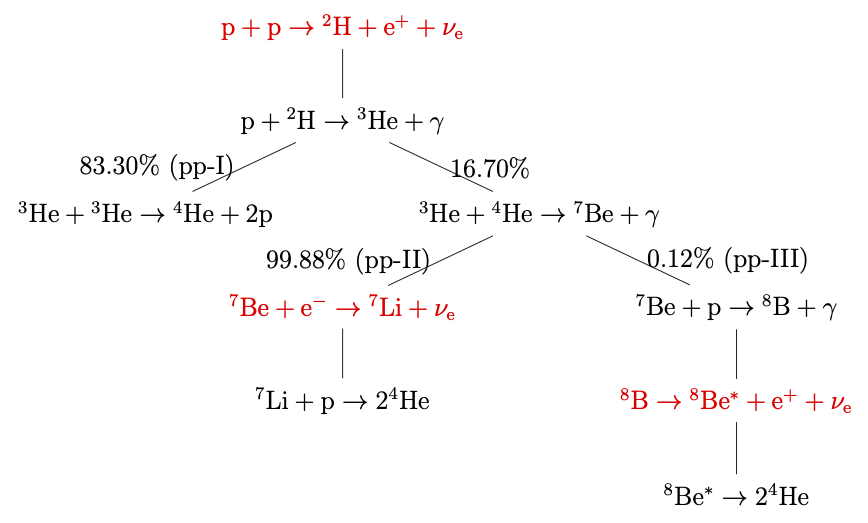# Stellar Neutrinos

Besides man-made sources, neutrinos are also produced in many astrophysical environments. For example, numerous nuclear reactions occur in stellar cores which produce luminous neutrino fluxes. The most important nuclear reactions in the Sun are the pp-chain reactions which are shown in Fig. pp Chain Branching.1 In order to calculate the neutrino spectrum one needs the neutrino production rate and the branching ratios for each reaction. Solar neutrinos are mostly produced in the pp reaction, Be electron capture and B decay which are labeled in red in Fig pp Chain Branching: \begin{align*} &\mathrm{p+p\to {}^2H + e^+ +\nu_e} & \mathrm{\leq 0.422MeV},\\ &\mathrm{{}^7Be + e^- \to {}^7Li + \nu_e} &\text{0.862MeV for 90\%},\\ &&\qquad \text{0.384MeV for 10\%}, \\ &\mathrm{{}^8B \to {}^8Be^* +e^+ +\nu_e} & \mathrm{\leq 15 MeV}. \end{align*}pp Chain BranchingThe pp chain reactions with the corresponding branching ratios. The branching ratios are taken from Ref [^Altmann2001].

Even without the knowledge of the detailed reactions, the conservation of the electric charge and the electron lepton number will lead to the overall neutrino production formula $$\begin{equation} \mathrm{4p+2e^- \to {}^4He + 2\nu_e }. \end{equation}$$ It is important to notice that two neutrinos are emitted for each $\alpha$ particle, i.e., ${}^4\mathrm{He}$, produced in the Sun. Using this simple relation, one can estimate the neutrino number flux emitted by the Sun. The energy released during the production of each $\alpha$ particle is the difference between the initial and final rest masses of the particles, $$\begin{equation} Q=4m_p+2m_e-m_{\alpha}=26.7\mathrm{MeV}, \end{equation}$$ where the masses of the neutrinos are neglected. On average, each neutrino carries away an amount of energy of $0.2\mathrm{MeV}$ and the rest of the energy is in the form of thermal energy $Q_\gamma=26.3\mathrm{MeV}$ 2. Since two neutrinos are emitted for the production of thermal energy $Q_\gamma$, the number flux of the solar neutrinos near the Earth is $$\begin{equation} \Phi_\nu = \frac{2 S_0}{Q_\gamma} \approx 6\times 10^{10} \mathrm{cm^{-2}s^{-1}}, \end{equation}$$ where the solar constant $S_0$ is the energy flux of solar photons on the top of the Earth atmosphere. It is a large amount of neutrinos released by the Sun.

As the detection of neutrinos became feasible, Ray Davis and John Bahcall et al. worked out the solar neutrino flux and led the Homestake experiment to measure the solar neutrinos. The results revealed that the neutrino flux detected was less than what was predicted by the standard solar model 3. This is the solar neutrino problem. It is now known that the solution to the problem is related to the neutrinos themselves. Some of the electron neutrinos produced in the solar core have transformed to other flavors before they eached the Earth. This phenomenon is referred as the flavor transformation of the neutrino, or neutrino oscillations. The theory of neutrino oscillations was first proposed by Pontecorvo in 1968 4. The field of neutrino oscillations has grown significantly into a broad field in physics since then.

1. Michael F Altmann, "Solar neutrinos", Reports on Progress in Physics 64, 97-146 (2001) . ↩︎

2. Eg Adelberger, "Solar fusion cross sections. II. The pp chain and CNO cycles", Reviews of Modern Physics 83, (2011) . ↩︎

3. J.N. Bahcall, "The solar neutrino problem", Nuclear Instruments and Methods 110, 381-384 (1973) . ↩︎

4. B Pontecorvo, "Neutrino experiments and the problem of conservation of leptonic charge", Soviet Physics JETP 26, 984-988 (1968) . ↩︎